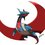# ya(n) failed, what about ya_2(n).

view my set. Lets recall $Aa(n)=\begin{cases} 1&,\text{if n is prime}\\0&,\text{otherwise}\end{cases}$.\ we define a new function: $ya_2(n)=(Aa*|\mu|)(n)=\sum_{p|n} |\mu(n/p)|$ We again make 3 cases

1.n is square free. we easily have $ya_2(n)=\omega(n)|\mu(n)|$

2.$n=p_1p_2p_3.....p_k^2$. in that case möbius will be zero everywhere except prime divisor bieng $p_k$ in which case it will becom |-1|=1. so the summation will be $ya_2(n)=1$

1. $ya_2(n)=0$ otherwise.

more formally $ya_2(n)=\begin{cases} \omega(n)|\mu(n)|&,\text{n is square free}\\1 &, \text{n has one squared prime factor}\\0 &,\text{otherwise}\end{cases}$ yes! this is great actually. convoluting both sides with $\lambda$ we have $Aa=ya_2*\lambda$ $\sum_{n=1}^\infty \dfrac{Aa(n)}{n^s}=\sum_{n=1}^\infty \dfrac{ya_2(n)}{n^s}\sum_{n=1}^\infty \dfrac{\lambda(n)}{n^s}$ $P(s)=\dfrac{\zeta(2s)}{\zeta(s)}\sum_{n=1}^\infty \dfrac{ya_2(n)}{n^s}$ We will compute $\sum_{n=1}^\infty \dfrac{Aa(n)}{n^s}$ in the next note.

dirichlet series and dirichlet convolution used here.Note by Aareyan Manzoor
4 years, 1 month ago

This discussion board is a place to discuss our Daily Challenges and the math and science related to those challenges. Explanations are more than just a solution — they should explain the steps and thinking strategies that you used to obtain the solution. Comments should further the discussion of math and science.

When posting on Brilliant:

• Use the emojis to react to an explanation, whether you're congratulating a job well done , or just really confused .
• Ask specific questions about the challenge or the steps in somebody's explanation. Well-posed questions can add a lot to the discussion, but posting "I don't understand!" doesn't help anyone.
• Try to contribute something new to the discussion, whether it is an extension, generalization or other idea related to the challenge.

MarkdownAppears as
*italics* or _italics_ italics
**bold** or __bold__ bold
- bulleted- list
• bulleted
• list
1. numbered2. list
1. numbered
2. list
Note: you must add a full line of space before and after lists for them to show up correctly
paragraph 1paragraph 2

paragraph 1

paragraph 2

[example link](https://brilliant.org)example link
> This is a quote
This is a quote
    # I indented these lines
# 4 spaces, and now they show
# up as a code block.

print "hello world"
# I indented these lines
# 4 spaces, and now they show
# up as a code block.

print "hello world"
MathAppears as
Remember to wrap math in $$ ... $$ or $ ... $ to ensure proper formatting.
2 \times 3 $2 \times 3$
2^{34} $2^{34}$
a_{i-1} $a_{i-1}$
\frac{2}{3} $\frac{2}{3}$
\sqrt{2} $\sqrt{2}$
\sum_{i=1}^3 $\sum_{i=1}^3$
\sin \theta $\sin \theta$
\boxed{123} $\boxed{123}$

Sort by:

Since these two functions are so similar, I'm guessing we will run into the same problem if we don't change the method we are using.

Here's what I can get using previous method (in part 2)

$\sum _{n=1}^{ \infty}\frac{ya_2\left(n\right)}{n^s}=\sum_{n=1}^{\infty}\frac{|\mu(n)|\omega(n)}{n^s}+\sum _{p\ \text{ prime}}^{ }\sum _{p\not\mid n}\frac{|\mu(n)|}{p^{2s}n^s}$

$\sum _{p\ \text{ prime}}^{ }\sum _{p\not\mid n}\frac{|\mu(n)|}{p^{2s}n^s}=\frac{\zeta(s)}{\zeta(2s)}\left(P\left(s\right)-\sum _p^{ }\frac{1}{p^s+1}\right) **$

For the second sum, I suggest trying to evaluate:

$\sum _{p\ \text{ prime}}^{ }\sum _{p\not\mid n}\frac{|\mu(n)|}{p^{2s}n^s}=\sum_{n=1}^{\infty}\frac{|\mu(n)|}{n^s}\sum_{p\mid n}\frac{1}{p^s}=\sum_{n=1}^{\infty}\frac{|\mu(n)|}{n^s}\sum_{p\not\mid n}\frac{1}{p^{2s}}**$

Also from methods in part 2,

$\sum_{n=1}^{\infty}\frac{|\mu(n)|}{n^s}\sum_{p\mid n}\frac{1}{p^s}=\frac{P(2s)\zeta(s)}{\zeta(2s)}-\sum_{n=1}^{\infty}\frac{|\mu(n)|}{n^s}\sum_{p\mid n}\frac{1}{p^{2s}}$

Those with $**$ have been verified numerically.

For the last equation, I am not sure if it matches numerically but it most probably is.

- 4 years ago

The second part was rather easy. my main concern is the first. i have found that $\omega|\mu|*1=\dfrac{\omega (|\mu|*1)}{2}$ which is kind of cool.

- 4 years ago

I'm getting

$\omega|\mu|*1(n)=\sum_{k=0}^{\omega(n)}k\left( \begin{matrix}\omega(n) \\ k \end{matrix} \right) =2^{\omega(n)-1}\omega(n)$

Going by the same logic in the second note,

$\omega|\mu|*\mu(n)=\sum_{k=0}^{\omega(n)}k*(-1)^{\omega(n)-k}\left( \begin{matrix}\omega(n) \\ k \end{matrix} \right)=\begin{cases} 1 \text{ if }n=p^a \\ 0 \text{ otherwise} \end{cases}$

Assuming what I did above is correct, I should get:

$\frac{1}{\zeta(s)}\sum_{n=1}^{\infty}\frac{|\mu(n)|\omega(n)}{n^s}=\sum_p\frac{1}{p^s-1}$

Again, the working above has not been verified numerically.

EDIT: Oh yeah, $|\mu|*1=2^{\omega(n)}$

EDIT again: Also, using the fact that

$\frac{1}{x+1}=\frac{1}{x-1}-\frac{2}{x^2-1}$

$\sum_p\frac{1}{p^s+1}=\sum_p\frac{1}{p^s-1}-\sum_p\frac{2}{p^{2s}-1}$

- 4 years ago

how do you always find a good function to convolute with! Lets see evaluating this $\omega|\mu|=L*1$ where L is the function you defined. so: $\sum_{n≥1} \dfrac{\omega|\mu|}{n^s}=\zeta(s)\sum_{prime} \dfrac{1}{p^s-1}$ Edit didnt see your edit.

- 4 years ago

Ah well Im stuck...

- 4 years ago

I think after evaluating we will get $P(s)=P(s)$ again.

- 4 years ago

I think so too, hopefully we don't

- 4 years ago

but something interesting is that $D(L;s)=\sum_p \dfrac{1}{p^s-1}$ and $L=\omega|\mu|*\mu=\omega\mu*1$ i think my target changed from finding an equation of primezeta to $D(L;s)$ after the second note.

- 4 years ago